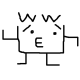How to move robot randomly while keeping on-arm camera locked at target.Hello guys.

Currently, I'm trying to reconstruct 3d model of object by using UR5, Realsense and AruCo. The idea is simple.

1. First, object is put on floor and surrounded by 4 Aruco tags (as below image) https://imgur.com/6P8QFHX
2. Secondly, we detected position of Aruco tags and convert into world cordinates. We also estimate the height of object roughly
3. Then robot will move at randomly position while the on-arm camera locked at the target and capturing point cloud at a fixed distance.
4. By using the cordinates and heights at 2nd steps, we crop the entire point cloud and get the point cloud of object-only. Then after few capture, we use the object-only point cloud to create the mesh.

For now, we are able to sample random position (x, y, z) for the camera. But we still don't know how to calculate the rotation of camera. In this image we draw each pose (position, rotation) on Rviz. We want the red arrow to point of object, but for now it's pointing at random location. https://imgur.com/gcxbSp1

Here is our piece of code used to sampling the pose (position + rotation):

def gen_acquisition_targets_from_sphere(self, center_pt, radius=0.4, sample_cnt=100, ndim=3):
""" Generating (pos, rot_q) pose for realsense to capture point cloud at fix radius using
"""
# https://stackoverflow.com/questions/33976911/generate-a-random-sample-of-points-distributed-on-the-surface-of-a-unit-sphere
# https://mathworld.wolfram.com/SpherePointPicking.html
# https://stackoverflow.com/questions/1251828/calculate-rotations-to-look-at-a-3d-point

# First generating a sample_cnt sample position
pts = np.random.randn(10000, ndim)
pts = (pts.T / np.linalg.norm(pts, axis=1)).T
# take the upper top only
indices = np.where(pts[:, 2] > 0.35)
pts = pts[indices]
pts = pts[0:sample_cnt, :]

# Calculate the Euler anglers
# We want the camera to point directly at object
poses = []
for i in range(np.shape(pts)):
x = pts[i, 0]
y = pts[i, 1]
z = pts[i, 2]

# direction vector to Euler angles
d = [-x, -y, -z]            # we want the camera to point at center point
tmp = math.sqrt(x*x + y*y + z*z)

ax = math.acos( -x / tmp)
ay = math.acos( -y / tmp)
az = math.acos( -z / tmp)

# https://www.intmath.com/vectors/7-vectors-in-3d-space.php

if (x > 0) and (y > 0):

rotx = ax
roty = ay
rotz = ay

# applying transformation
x = x * radius + center_pt
y = y * radius + center_pt
z = z * radius + center_pt

rot_q = quaternion_from_euler(rotx, roty, rotz)
pose = [
(x, y, z),
rot_q
]

poses.append(pose)

return poses
edit retag close merge delete